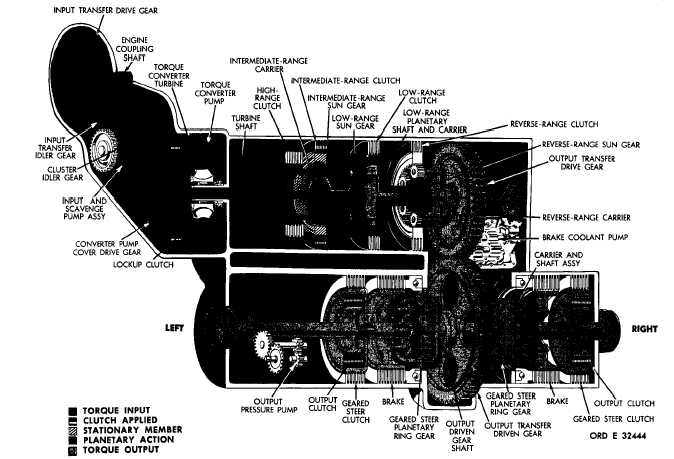Custom SearchC H A P    2,   SEC  V T O R Q U E     P A T HS P A R    4   9 Figure   25.   Third-gear   torque   path,   lockup   operation 49.   TORQUE   PATH   –   THIRD   GEAR, LOCKUP   OPERATION   (fig.   25) .   Torque   is   transmitted   to   the   low-   and intermediate-range   planetary   sun   gears   in   the manner   described   in   pars.   42   and   43,   above. In   third   gear,   the   low-   and   intermediate-range p l a n e t a r y    g e a r s    a r e    c o m p o u n d e d    t o    p r o d u ce the   desired   speed   reduction   ratio.   The   inter- mediate-range   clutch   is   engaged,   holding   the intermediate-range    ring    gear    stationary.    The intermediate-range    planetary    pinions,    meshed with   the   intermediate-range   sun   gear,   which is   rotating,   and   the   stationary   ring   gear,   must rotate   within   the   ring   gear.   This   action   drives t h e    i n t e r m e d i a t e - r a n g e    p l a n e t a r y    c a r r i e r. .   The   low-range   planetary   ring   gear   is attached   to   the   intermediate-range   carrier   and rotates   at   a   slower   speed   than   the   low-range sun   gear.   Thus,   the   faster   moving   sun   gear, and   slower   moving   ring   gear,   combine   to   drive the    low-range    planetary    pinions.    The    output member   of   this   compound   arrangement   is   the low-range   planetary   carrier   and   shaft. .   T h e    r e m a i n d e r    o f    t h e    t o r q u e    p a t h    is i d e n t i c a l    t o    t h a t    d e s c r i b e d    f o r    s e c o n d    g e ar ( p a r .    4 8 ,    a b o v e ) . Refer   to   T A B U L A T E D D A T A ,   par.   7,   for   gear   ratios   applicable   to third   gear. 52Integrated Publishing, Inc. - A (SDVOSB) Service Disabled Veteran Owned Small Business